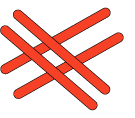#### You may also like### Pebbles

Place four pebbles on the sand in the form of a square. Keep adding as few pebbles as necessary to double the area. How many extra pebbles are added each time?### It Figures

Suppose we allow ourselves to use three numbers less than 10 and multiply them together. How many different products can you find? How do you know you've got them all?### Bracelets

Investigate the different shaped bracelets you could make from 18 different spherical beads. How do they compare if you use 24 beads?

# Crossings

## Crossings

In this problem we are looking at sets of parallel sticks that cross each other.There are four red sticks which make four crossings.
How many crossings do the five green sticks make?

Still keeping two sets of parallel sticks, this time with seven sticks in total, can you arrange them in another way, to get a different number of crossings?
What is the least number of crossings you can make?
What is the greatest number of crossings you can make?
Can you find all possible numbers of crossings with seven sticks?
What do you need to do to prove that you have them all or how could you show that you have them all?

Can you find the least/greatest number of crossings for ten sticks?
Can you find the least/greatest number of crossings for fifteen sticks?
Can you predict the least/greatest number of crossings for fifty sticks?
Can you predict the least/greatest number of crossings for any number of sticks?

### Why do this problem?

This problem gives learners a great opportunity to apply number facts and skills to a seemingly spatial problem. It is also a good context for them to give reasons for the patterns which emerge.

### Possible approach

Many could benefit from having sticks or straws with which to investigate this problem, although it can be tackled purely by sketching sticks.

### Key questions

What is the least number you may have in a "set of sticks"?

If you start with a set of $2$, how many sticks would be in the set placed across these $2$?
How many crossings would that make?

Can you think of a good way of recording what you have done?

Do you notice anything about the number of sticks in each set and the number of crossings?
How do these numbers relate to the total number of sticks?

If you have $10$ sticks how many could you put down to start with?
How many would be left to cross them?
How many crossings would this make?
Can you arrange them to make more/less crossings?

Can you generalise your ideas for any number?

### Possible extension

Learners could go on to Divide it out.

### Possible support

Suggest using sticks or straws to model the questions asked and then sketch what has been done.